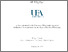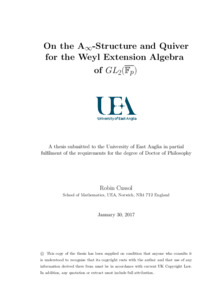# On the A_\infty-Structure and Quiver for the Weyl Extension Algebra of GL_2(\overline{F_p})

Cussol, Robin (2017) On the A_\infty-Structure and Quiver for the Weyl Extension Algebra of GL_2(\overline{F_p}). Doctoral thesis, University of East Anglia.Preview PDF Download (1MB) | Preview

## Abstract

This research belongs to the field of Representation Theory and tries to solve questions through homological algebraic methods. This project deals with the study of symmetries of the plane and aims at measuring how much a mathematical object of importance for that study fails to satisfy the property of not needing bracketing when multiplying three elements together, which is called associativity. More precisely, we study the rational representations of GL_2(\overline{F_p}), the general linear group of order 2 over an algebraically closed field of prime characteristic p. Representations are a means to understand group or algebra elements as linear transformations on a vector space of a given dimension, and it is possible to "build" representations from smaller ones, e.g. the set of so-called standard representations. The way to glue these building blocks together is governed by the algebra of extensions between standard representations. In a series of papers culminating with [MT13], Miemietz and Turner described precisely the algebra structure of that extension algebra. It is the homology of a differential-graded algebra and this project aims at estimating how non-associative it is by computing its A-infinity-algebra structure. For any p, we give the quiver of that extension algebra, and for p = 2, we show that there exists a subalgebra of the extension algebra which admits a trivial A-infinity-algebra structure, and what's more, in a somewhat peculiar way. We also give its quiver and discuss some of its properties.

Item Type: Thesis (Doctoral) Title and abstract amended on repository 2/6/17. Faculty of Science > School of Mathematics Users 4971 not found. 03 Mar 2017 14:40 02 Jun 2017 10:08 https://ueaeprints.uea.ac.uk/id/eprint/62848View Item